A quadratic equation is an equation that could be written as

ax 2 + bx + c = 0

when a 0.

There are three basic methods for solving quadratic equations: factoring, using the quadratic formula, and completing the square.

#### Factoring

To solve a quadratic equation by factoring,

1. Put all terms on one side of the equal sign, leaving zero on the other side.

2. Factor.

3. Set each factor equal to zero.

4. Solve each of these equations.

##### Example 1

Solve x 2 – 6 x = 16.

Following the steps,

x 2 – 6 x = 16 becomes x 2 – 6 x – 16 = 0

Factor.

( x – 8)( x + 2) = 0

Setting each factor to zero,Then to check,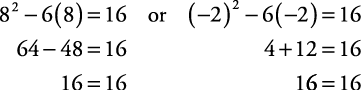Both values, 8 and –2, are solutions to the original equation.

##### Example 2

Solve y 2 = – 6 y – 5.

Setting all terms equal to zero,

y 2 + 6 y + 5 = 0

Factor.

( y + 5)( y + 1) = 0

Setting each factor to 0,To check, y 2 = –6 y – 5A quadratic with a term missing is called an incomplete quadratic (as long as the ax 2 term isn't missing).

##### Example 3

Solve x 2 – 16 = 0.

Factor.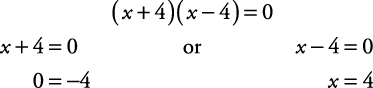To check, x 2 – 16 = 0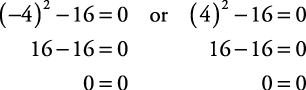##### Example 4

Solve x 2 + 6 x = 0.

Factor.To check, x 2 + 6 x = 0##### Example 5

Solve 2 x 2 + 2 x – 1 = x 2 + 6 x – 5.

First, simplify by putting all terms on one side and combining like terms.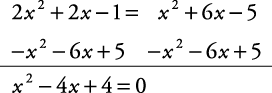Now, factor.To check, 2 x 2 + 2 x – 1 = x 2 + 6 x – 5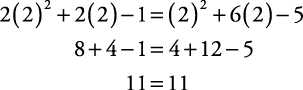Many quadratic equations cannot be solved by factoring. This is generally true when the roots, or answers, are not rational numbers. A second method of solving quadratic equations involves the use of the following formula: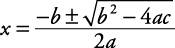a, b, and c are taken from the quadratic equation written in its general form of

ax 2 + bx + c = 0

where a is the numeral that goes in front of x 2, b is the numeral that goes in front of x, and c is the numeral with no variable next to it (a.k.a., “the constant”).

When using the quadratic formula, you should be aware of three possibilities. These three possibilities are distinguished by a part of the formula called the discriminant. The discriminant is the value under the radical sign, b 2 – 4 ac. A quadratic equation with real numbers as coefficients can have the following:

1. Two different real roots if the discriminant b 2 – 4 ac is a positive number.

2. One real root if the discriminant b 2 – 4 ac is equal to 0.

3. No real root if the discriminant b 2 – 4 ac is a negative number.

##### Example 6

Solve for x: x 2 – 5 x = –6.

Setting all terms equal to 0,

x 2 – 5 x + 6 = 0

Then substitute 1 (which is understood to be in front of the x 2), –5, and 6 for a, b, and c, respectively, in the quadratic formula and simplify.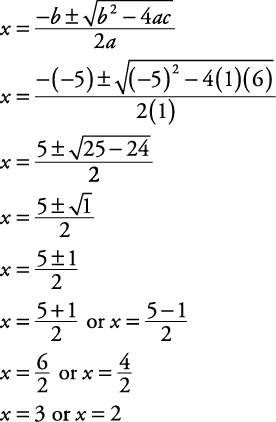Because the discriminant b 2 – 4 ac is positive, you get two different real roots.

Example produces rational roots. In Example , the quadratic formula is used to solve an equation whose roots are not rational.

##### Example 7

Solve for y: y 2 = –2y + 2.

Setting all terms equal to 0,

y 2 + 2 y – 2 = 0

Then substitute 1, 2, and –2 for a, b, and c, respectively, in the quadratic formula and simplify.Note that the two roots are irrational.

##### Example 8

Solve for x: x 2 + 2 x + 1 = 0.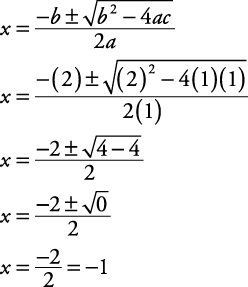Since the discriminant b 2 – 4 ac is 0, the equation has one root.

The quadratic formula can also be used to solve quadratic equations whose roots are imaginary numbers, that is, they have no solution in the real number system.

##### Example 9

Solve for x: x( x + 2) + 2 = 0, or x 2 + 2 x + 2 = 0.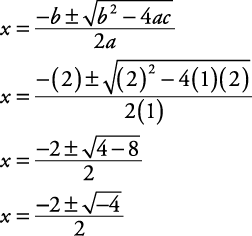Since the discriminant b 2 – 4 ac is negative, this equation has no solution in the real number system.

But if you were to express the solution using imaginary numbers, the solutions would be.

#### Completing the square

A third method of solving quadratic equations that works with both real and imaginary roots is called completing the square.

1. Put the equation into the form ax 2 + bx = – c.

2. Make sure that a = 1 (if a ≠ 1, multiply through the equation by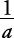before proceeding).

3. Using the value of b from this new equation, add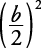to both sides of the equation to form a perfect square on the left side of the equation.

4. Find the square root of both sides of the equation.

5. Solve the resulting equation.

##### Example 10

Solve for x: x 2 – 6 x + 5 = 0.

Arrange in the form of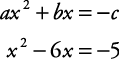Because a = 1, add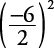, or 9, to both sides to complete the square.Take the square root of both sides.

x – 3 = ±2

Solve.##### Example 11

Solve for y: y 2+ 2 y – 4 = 0.

Arrange in the form ofBecause a = 1, add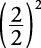, or 1, to both sides to complete the square.Take the square root of both sides.Solve.##### Example 12

Solve for x: 2 x 2 + 3 x + 2 = 0.

Arrange in the form of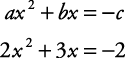Because a ≠ 1, multiply through the equation by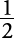.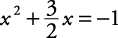Addorto both sides.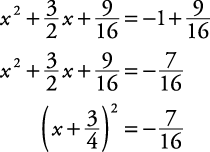Take the square root of both sides.There is no solution in the real number system. It may interest you to know that the completing the square process for solving quadratic equations was used on the equation ax 2 + bx + c = 0 to derive the quadratic formula.